# Logical Reasoning and Analytical Ability (Test 1)

## Upsc Civil Services Entrance Exams : General Studies Test

| Home | | Upsc Civil Services Entrance Exams | | General Studies Test | | Logical Reasoning and Analytical Ability |Logical Reasoning and Analytical Ability
| Logical Reasoning | | Analytical Reasoning |
Q.1
Find the number of triangles in the given figure.A. 8
B. 10
C. 12
D. 14
Answer : Option D
Explaination / Solution:

The figure may be labelled as shown.The simplest triangles are AHG, AIG, AIB, JFE, CJE and CED i.e. 6 in number.

The triangles composed of two components each are ABG, CFE, ACJ and EGI i.e. 4 in number.

The triangles composed of three components each are ACE, AGE and CFD i.e. 3 in number.

There is only one triangle i.e. AHE composed of four components.

Therefore, There are 6 + 4 + 3 + 1 = 14 triangles in the given figure.

Workspace
Report
Q.2
Find the minimum number of straight lines required to make the given figure.A. 13
B. 15
C. 17
D. 19
Answer : Option A
Explaination / Solution:

The figure may be labelled as shown.The horizontal lines are IJ, AB, EF, MN, HG, DC and LK i.e. 7 in number.

The vertical lines are AD, EH, IL, FG, BC and JK i.e. 6 in number.

Thus, there are 7 + 6 = 13 straight lines in the figure.

Workspace
Report
Q.3
Find the number of triangles in the given figure.A. 21
B. 23
C. 25
D. 27
Answer : Option D
Explaination / Solution:

The figure may be labelled as shown.The simplest triangles are ABL, BCD, DEF, FGP, PGH, QHI, JQI, KRJ and LRK i.e. 9 in number.

The triangles composed of two components each are OSG, SGQ, SPI, SRI, KSQ, KMS, FGH, JHI and JKL i.e. 9 in number.

There is only one triangle i.e. KSG which is composed of four components.

The triangles composed of five components each are NEI, ANI, MCG and KCO i.e. 4 in number.

The triangles composed of six components each are GMK and KOG i.e. 2 in number.

There is only one triangle i.e. AEI composed of ten components.

There is only one triangle i.e. KCG composed of eleven components.

Therefore, Total number of triangles in the given figure = 9 + 9+1 + 4 + 2+1 + 1 = 27.

Workspace
Report
Q.4

Find the number of triangles in the given figure.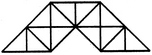A. 23
B. 27
C. 29
D. 31
Answer : Option C
Explaination / Solution:

The figure may be labelled as shown.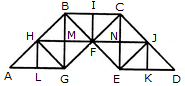The simplest triangles are AHL, LHG, GHM, HMB, GMF, BMF, BIF, CIF, FNC, CNJ, FNE, NEJ, EKJ and JKD i.e. 14 in number.

The triangles composed of two components each are AGH, BHG, HBF, BFG, HFG, BCF, CJF, CJE, JEF, CFE and JED i.e. 11 in number.

The triangles composed of four components each are ABG, CBG, BCE and CED i.e. 4 in number.

Total number of triangles in the given figure = 14 + 11 + 4 = 29.

Workspace
Report
Q.5

Find the number of triangles in the given figure.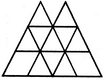A. 16
B. 18
C. 14
D. 15
Answer : Option B
Explaination / Solution:

The figure may be labelled as shown.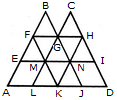The simplest triangles are BFG, CGH, EFM, FMG, GMN, GHN, HNI, LMK, MNK and KNJ i.e. 10 in number.

The triangles composed of three components each are FAK and HKD i.e. 2 in number.

The triangles composed of four components each are BEN, CMI, GLJ and FHK i.e. 4 in number.

The triangles composed of eight components each are BAJ and OLD i.e. 2 in number.

Thus, there are 10 + 2 + 4 + 2 = 18 triangles in the given figure.

Workspace
Report
Q.6

Find the number of triangles in the given figure.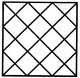A. 28
B. 32
C. 36
D. 40
Answer : Option C
Explaination / Solution:

The figure may be labelled as shown.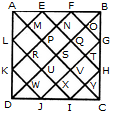The simplest triangles are AML, LRK, KWD, DWJ, JXI, IYC, CYH, HTG, GOB, BOF, FNE and EMA i.e. 12 in number.

The triangles composed of two components each are AEL, KDJ, HIC and FBG i.e. 4 in number.

The triangles composed of three components each are APF, EQB, BQH, GVC, CVJ, IUD, DUL and KPA i.e. 8 in number.

The triangles composed of six components each are ASB, BSC, CSD, DSA, AKF, EBH, CGJ and IDL i.e. 8 in number.

The triangles composed of twelve components each are ADB, ABC, BCD and CDA i.e. 4 in number.

Total number of triangles in the figure = 12 + 4 + 8 + 8 + 4 = 36.

Workspace
Report
Q.7
Find the number of triangles in the given figure.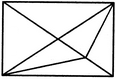A. 11
B. 13
C. 15
D. 17
Answer : Option C
Explaination / Solution:

The figure may be labelled as shown.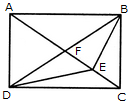The simplest triangles are AFB, FEB, EBC, DEC, DFE and AFD i.e. 6 in number.

The triangles composed of two components each are AEB, FBC, DFC, ADE, DBE and ABD i.e. 6 in number.

The triangles composed of three components each are ADC and ABC i.e. 2 in number.

There is only one triangle i.e. DBC which is composed of four components.

Thus, there are 6 + 6 + 2 + 1 = 15 triangles in the figure.

Workspace
Report
Q.8

Count the number of triangles and squares in the given figure.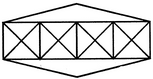A. 36 triangles, 7 squares
B. 38 triangles, 9 squares
C. 40 triangles, 7 squares
D. 42 triangles, 9 squares
Answer : Option C
Explaination / Solution:

The figure may be labelled as shown.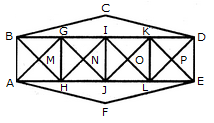Triangles:

The simplest triangles are BGM, GHM, HAM, ABM, GIN, UN, JHN, HGN, IKO, KLO, LJO, JIO, KDP, DEP, ELP, LKP, BCD and AFE i.e. 18 in number.

The triangles composed of two components each are ABG, BGH, GHA, HAB, HGI, GIJ, IJH, JHG, JIK, IKL, KLJ, LJI, LKD, KDE, DEL and ELK i.e. 16 in number.

The triangles composed of four components each are BHI, GJK, ILD, AGJ, HIL and JKE i.e. 6 in number.

Total number of triangles in the figure = 18 +16 + 6 = 40.

Squares :

The squares composed of two components each are MGNH, NIOJ and OKPL i.e. 3 in number.

The squares composed of four components each are BGHA, GIJH, IKLJ and KDEL i.e. 4 in number.

Total number of squares in the figure = 3 + 4 = 7.

Workspace
Report
Q.9
In the adjoining figure, if the centres of all the circles are joined by horizontal and vertical lines, then find the number of squares that can be formed.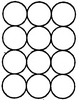A. 6
B. 7
C. 8
D. 1
Answer : Option C
Explaination / Solution:

The figure may be labelled as shown.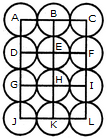We shall join the centres of all the circles by horizontal and vertical lines and then label the resulting figure as shown.

The simplest squares are ABED, BCFE, DEHG, EFIH, GHKJ and HILK i.e. 6 in number.

The squares composed of four simple squares are ACIG and DFLJ i.e. 2 in number.

Thus, 6 + 2 = 8 squares will be formed.

Workspace
Report
Q.10

Count the number of parallelogram in the given figure.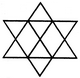A. 8
B. 11
C. 12
D. 15
Answer : Option D
Explaination / Solution:

The figure may be labelled as shown.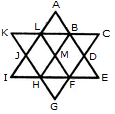The simplest parallelograms are LMHJ and BDFM i.e. 2 in number. The parallelograms composed of two components each are ABML and MFGH i.e. 2 in number.

The parallelograms composed of three components each are LBHI, LBEF, BDGH, DFLA, BCFH, KLFH, A6HJ and LFGJ i.e. 8 in number.

The parallelograms composed of six components each are LCFI, KBEH and ADGJ i.e. 3 in number.

Total number of parallelograms in the figure = 2 + 2 + 8 + 3 = 15.

Workspace
Report